# What is standard form?

Standard form helps us represent numbers and equations clearly and consistently. Let’s explore the concept in more detail!Author
Michelle GriczikaPublished
Sep 22nd, 2023# What is standard form?

Standard form helps us represent numbers and equations clearly and consistently. Let’s explore the concept in more detail!Author
Michelle GriczikaPublished
Sep 22nd, 2023# What is standard form?

Standard form helps us represent numbers and equations clearly and consistently. Let’s explore the concept in more detail!Author
Michelle GriczikaPublished
Sep 22nd, 2023Key takeaways

• Standard form is a concept that helps us to consistently represent numbers and equations
• Fractions and equations can be written in standard forms

Standard form is an important mathematical concept that helps us represent numbers and equations clearly and consistently. It may sound a bit technical, but don’t worry! In this article, we’ll explore standard form in a simple and clear way. Let’s get started!

## What is standard form?

Let’s start by understanding what standard form really means.

Standard form is a special way of writing numbers or equations that helps us easily compare and work with them

In standard form, numbers are written as a multiplication of two parts: a number between 1 and 10 and a power of 10. Let’s break it down with some examples.

## How to write numbers in standard form

To write numbers in standard form, we follow a simple process.

First, we identify the number between 1 and 10 and then determine how many times we need to multiply it by 10 to get the original number.

Let’s take the number 5,000 as an example. We can write it in standard form as 5 x 10^3. The number 5 is between 1 and 10, and we multiply it by 10 three times to get 5,000.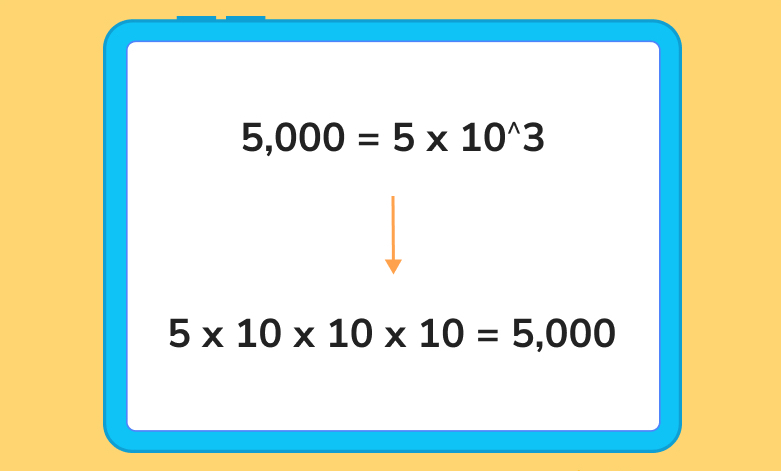## Advanced topics in standard form

Now, let’s explore some advanced topics in standard form. Don’t worry if it seems a little challenging at first; understanding these concepts will help you as you progress in your mathematical journey.

### Standard form of fractions

You can use standard form with fractions. When dealing with fractions, we can write the numerator and denominator in standard form separately.

Here’s one example of standard format: the fraction 3/20 can be expressed as 3 x 10^0 / 2 x 10^1. When we simplify it, we get 1.5 x 10^-1. It’s like magic!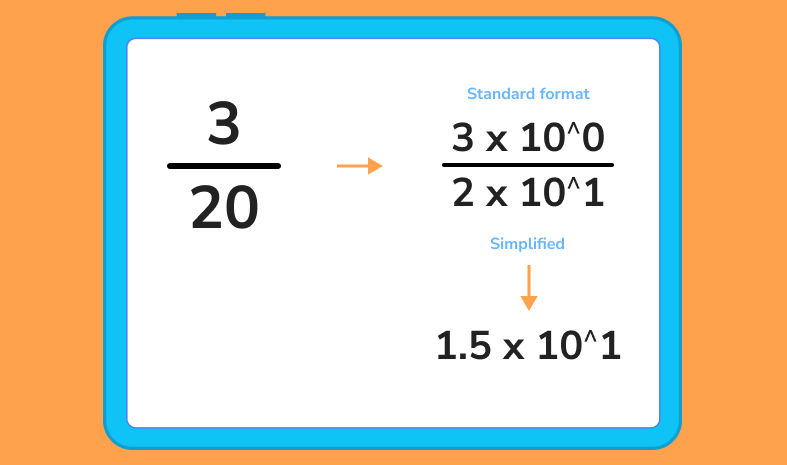## Explore standard form with DoodleMaths

DoodleMaths is an award-winning app that’s filled with fun, interactive questions exploring standard form and beyond!

Created by teachers, DoodleMaths creates every child a personalised learning experience tailored to their needs, boosting their confidence and ability in maths.

Designed to be used for 10 minutes a day, it automatically targets tricky topics, tops up knowledge and introduces new areas, helping kids get ahead in their learning. Try it for free today!### Standard form of equations

Equations can also be written in standard form. Let’s start with linear equations.

In standard form, a linear equation looks like this: Ax + By = C. The letters A, B, and C represent numbers we need to find.

Quadratic equations also have a standard form, written as Ax^2 + Bx + C = 0.

#### Linear equation standard form

The standard form is represented in linear equations as Ax + By = C, where A, B, and C are constants. This form clearly lets us see the coefficients (the numbers multiplying x and y).

For example, the equation 2x + 3y = 7 is in standard form. Therefore, we can easily compare the coefficients by writing equations in this form and see how x and y are related!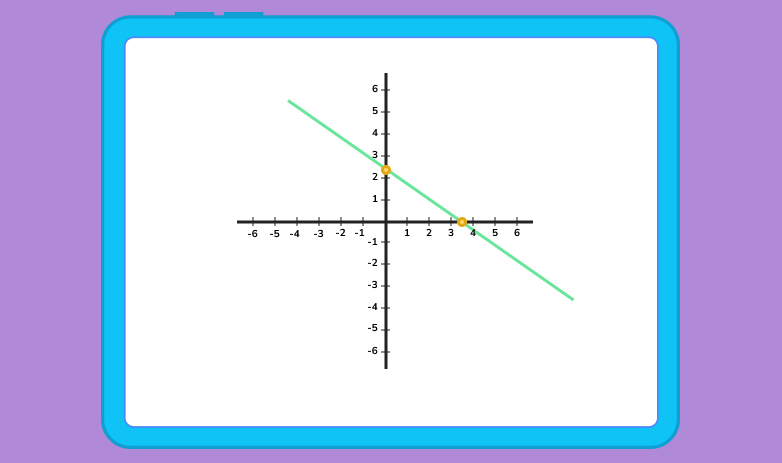Quadratic equations also have a standard form, written as Ax^2 + Bx + C = 0. This form helps us identify the quadratic, linear, and constant coefficients.

For instance, the equation x^2 – 5x + 6 = 0 is in standard form. By expressing quadratic equations this way, we can quickly recognise the coefficients and understand the behaviour of the quadratic function.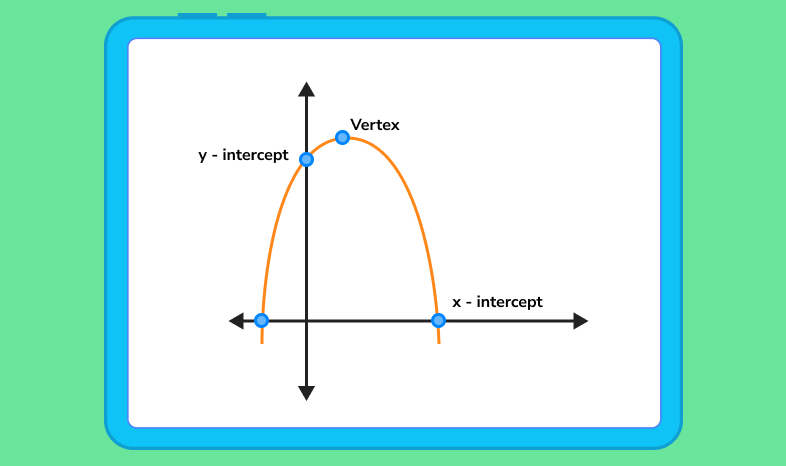### Standard form of polynomials

You can write polynomials with multiple terms in standard form, too. We arrange the terms in descending order of their degree, which means the highest power comes first, followed by the others.

For example, the polynomial 5x^2 – 2x^3 + 3x^4 – 7x + 1 can be written in standard form as 3x^4 – 2x^3 + 5x^2 – 7x + 1.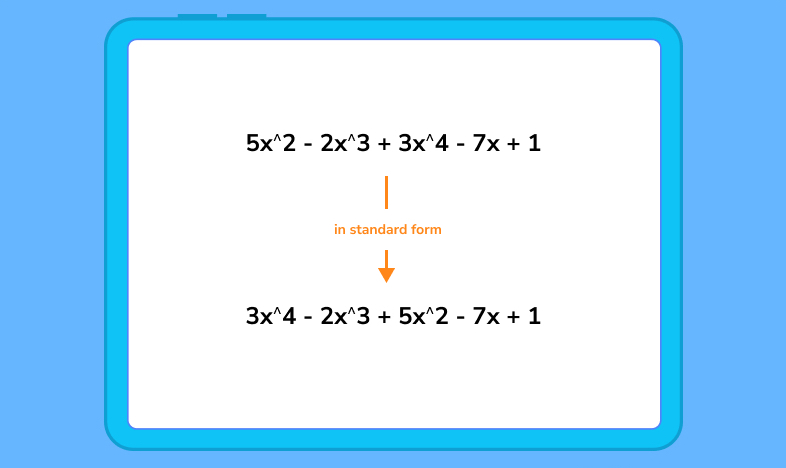Standard form in maths refers to representing numbers or equations in a consistent format that includes a coefficient and a power of 10.

An example of standard form is 4.2 x 10^2, which represents the number 420.

Yes, standard form and scientific notation are the same concepts! Both involve writing numbers as a coefficient multiplied by a power of 10.### In summary...

Congratulations! You’ve now gained a solid understanding of standard form — a powerful tool in maths.

Remember, standard form is all about breaking down numbers and equations into parts that are easy to understand and work with. Whether writing numbers in standard form or expressing fractions, standard form simplifies how we communicate and solve mathematical problems.

If you want to further enhance your skills, be sure to check out the DoodleMaths app. It’s filled with engaging activities and exercises that will help reinforce your understanding of standard form and many other mathematical concepts!Lesson credits

Michelle Griczika

Michelle Griczika is a seasoned educator and experienced freelance writer. Her years teaching first and fifth grades coupled with her double certification in elementary and early childhood education lend depth to her understanding of diverse learning stages. Michelle enjoys running in her free time and undertaking home projects.

Michelle Griczika

Michelle Griczika is a seasoned educator and experienced freelance writer. Her years teaching first and fifth grades coupled with her double certification in elementary and early childhood education lend depth to her understanding of diverse learning stages. Michelle enjoys running in her free time and undertaking home projects.

## Related posts

Rational vs irrational numbers

Learn about the differences between rational and irrational numbers

What are odd and even numbers?

We explore examples of odd and even numbers from 1 to 100

Roman numerals from 1 to 100

Learn the Roman numerals from 1 to 100 and have a go at some questions!

# Are you a parent, teacher or student?

Are you a parent or teacher?

## Hi there!

Book a chat with our team